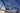# Loops in Python Explained

Tue Aug 04 2020## Loop with index

The way we do it here is using the indexes and getting the item with the index each time.

``````x = [1,3,5,7,9]
sum_squared = 0

for i in range(len(x)):
sum_squared+=x[i]**2``````

## Loop with actual value

here we skip the index, of course this works only in the case we don't need the index.

``````x = [1,3,5,7,9]
sum_squared = 0

for y in x:
sum_squared+=y**2``````

## One Liner

Here we loop over the array and in the same line power the value

``````x = [1,3,5,7,9]
sum_squared = sum([y**2 for y in x])``````

## Inline Loop with if

Same as above, but with small if to filter the values.

``````x = [1,2,3,4,5,6,7,8,9]
even_squared = [y**2 for y in x if y%2==0]
--------------------------------------------
[4,16,36,64]``````

## Inline Loop with if/else

Now we use if/else in case we want to handle the mapping in a different way by the value.

``````x = [1,2,3,4,5,6,7,8,9]
squared_cubed = [y**2 if y%2==0 else y**3 for y in x]
--------------------------------------------
[1, 4, 27, 16, 125, 36, 343, 64, 729]``````